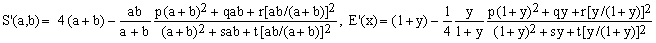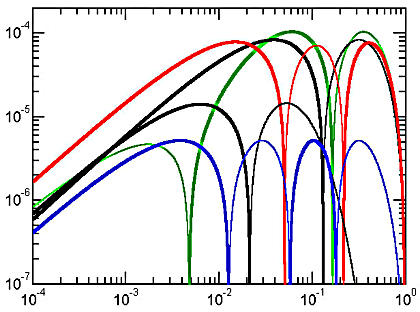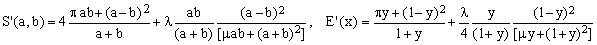Approximations of Ellipse Perimeters and of the Complete Elliptic Integral E(x) Addendum to the 2005 Review by Stanislav Sýkora, Extra Byte, Via R.Sanzio 22C, Castano Primo, Italy 20022 in Stan's Library, Ed.S.Sykora, Vol.I. First release February 20, 2006 Permalink via DOI:  10.3247/SL1Math05.004add
 Other math articles in Stan's Library | SCIENCE Links Stan's Courses | Stan's HUB
 This document updates the 2005 Review of Approximations of Ellipse Perimeters. Readers who are not acquainted with the topic should consult the original review before reading this document. Note added on June 6, 2006:  Algebraic Approximations of Ellipse Perimeters, a new category with several novel entries. Note added on March 15, 2007:  Advances in Approximations of Ellipse Perimeters with a new class (S) of approximations, several comments, and a new formula (optimized Ahmadi) which has a maximum relative error of just 152 ppb. Note added on August 1, 2007:  A historic comment on ellipse perimeter approximations.
 Introduction |  The new approximation |  Various comments |  Next goal |  References and links
 Introduction Barely two months have passed since the publication of my Review (hence just R) on the Approximations of Ellipse Perimeters and I already feel compelled to amend it. There are two specific reasons for this: (1) I have concluded R with the following challenge: Those who wish to pursue this topic further should look for an efficient formula using only the four algebraic operations (if possible, avoiding even square-root) with a maximum error below 10 ppm. If would be also nice if such a formula were exact for both the circle (y=1) and the degenerate flat ellipse (y=0). Writing those words, I certainly did not expect that in less than one month David W.Cantrell would come up with an approximation that meets all those criteria and has the maximum relative error of just 5.2 ppm !!! (2) David, Gérard P.Michon and I have exchanged several e-mails which clarified an issue of priority, plus some minor points which are worth mentioning. The new approximation (C7) Cantrell 2006 The new approximation is exact for both y = 1 (circle) and y = 0 (degenerate, flat ellipse) and has three zero crossings, i.e., points at which S'(a,b) = S(a,b). According to the classification of the original Review, it therefore belongs to category C and becomes its seventh and most successful member. The new formulae, originating from an artful combination of arithmetic, geometric and harmonic means, are: (C7), where p, q, r, s and t are numeric parameters. For the formula to be exact at y=0, r must equal 4[(4-π)(4s+t+16)-(4p+q)], leaving four parameters to be adjusted so as to minimize the maximum relative error. The resulting optimal values are p = 3.982901, q = 66.71674, s = 18.31287, t = 23.39728 and r = 56.2007. Notice that, due to the repetition of many factors occurring in Eqs.(C7), the evaluation of the formula is computationally quite efficient. Cantrell shows that there is an extended lower-dimensional region in the space of the parameters {p,q,s,t} in which the maximum relative error remains close to its best value of 5.2 ppm. This is reminiscent of fitting transcendental functions by high-order polynomials where one also finds extensive regions of parameter combinations within which the fits remain quite satisfactory. The error curve of the new approximation is shown in blue color in the Figure below. For comparison, the Figure shows the error curves for five most successful approximations found so far (excluding the Padé combinations discussed in R).Error curves for the best approximations with exact extremes  In order of decreasing maximum relative error, the approximations are: (R:C3, green) Rivera II, (R:C4, black) Cantrell I, (R:C5, red) Sykora, (R:C6, black) Cantrell-Ramanujan, and (C7, blue) Cantrell 2006. The thin lobes of each curve correspond to negative deviations with S'(a,b)<=S(a,b) and the thick ones to non-negative deviations. Approximations (R:C5) and (C7) are the only ones containing only basic arithmetic operations.   Various comments The following comments stem from discussions between me and David Cantrell, whom I thank for his help and friendly advice. Priority claims for (R:E5) When writing my first article on ellipse perimeters, I was convinced that (R:E5) was quite original. Soon after the article went online, however, I have found out that the formula matches the first term of D.F.Rivera's approximation (R:C3). I have therefore amended the article with a note to that effect and, in the Review, coined the term Sykora-Rivera approximation. Then, reading the article and the Review, David Cantrell realized that an earlier expression of his which he called particularly fruitful, though written differently, is identical to mine and Rivera's. Mentioning the fact to me, he added: I doubt that I am the first person to have suggested using (R:E5) to approximate the perimeter of an ellipse. It's such a simple approximation that it was probably suggested long ago by someone else. But who? I therefore suggest that (R:E5) be henceforth called Cantrell's Particularly Fruitful approximation. Optimization of (R:C5) This formula originates from my first article where it has been cast in a more general form (Eqs.9-10) containing two adjustable parameters whose optimal values, λ = -0.50079... and μ = 3.1524..., give maximum relative error of 76 ppm. Intrigued by the fact that these optimal values are so close to the esthetically pleasing λ = -1/2 and μ = π, I have opted for the latter despite the fact that this leads to a slightly larger error (78.5 ppm). Since David disagrees with such numerological mysticism, I list here the optimized form of (R:C5), leaving it to the readers to decide whether they like it more or not: (R:C5, optimal), with the optimal values of λ and μ given above. Matters of numeric efficiency Throughout the Review, I have often mentioned computational efficiency of the approximation formulae, vaguely related to the minimum number of arithmetic operations required to evaluate them. However, I have never attempted to assess the efficiency in a quantitative way. Algorithmic optimization of expressions is a non-trivial task (it may even require taking into account the hardware on which to perform the calculations) and I felt that, though relevant, it was not the most important aspect of the subject matter. Next goal The only possible way to conclude this Addendum is by re-iterating the original challenge with more stringent numeric limits: Those who wish to pursue this topic further should look for an efficient formula using only the four algebraic operations (if possible, avoiding even square-root) with a maximum error smaller than 1 ppm. In addition, the formula should be exact for both the circle (y=1) and the degenerate flat ellipse (y=0).
 References Sykora S., Approximations of Ellipse Perimeters: Addendum, in Stan's Library, S.Sykora, Vol I, 2006. (URL of this Note). Sykora S., Approximations of Ellipse Perimeters, in Stan's Library, S.Sykora, Vol I, 2005. The original Review denoted as R.  For more references, click here. Web links Two New Approximations, in a Certain Form, for the Perimeter of an Ellipse by David W. Cantrell. Arithmetic Approximations of the Perimeter of an Ellipse by David W. Cantrell.  For more links, click here.
 TOP | Other math articles in Stan's Library | SCIENCE Links Stan's Courses | Stan's HUB | TOP